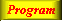Lecture 3 - Page 12 : 42
 Functional Programming in SchemeName binding, Recursion, Iteration, and Continuations * Name binding constructs The let name binding expression The equivalent meaning of let Examples with let name binding The let* name binding construct An example with let* The letrec namebinding construct LAML time functions * Conditional expressions Conditional expressions Examples with if Example with cond: leap-year? Example with cond: american-time Example with cond: as-string * Recursion and iteration Recursion List processing Tree processing (1) Tree processing (2) Recursion versus iteration Example of recursion: number-interval Examples of recursion: string-merge Examples with recursion: string-of-char-list? Exercises * Example of recursion: Hilbert Curves Hilbert Curves Building Hilbert Curves of order 1 Building Hilbert Curves of order 2 Building Hilbert Curves of order 3 Building Hilbert Curves of order 4 A program making Hilbert Curves * Continuations Introduction and motivation The catch and throw idea A catch and throw example The intuition behind continuations Being more precise The capturing of continuations Capturing, storing, and applying continuations Use of continuations for escaping purposes Practical example: Length of an improper list Practical example: Searching a binary tree
 Example with cond: leap-year?
 ```(define (leap-year? y) (cond ((= (modulo y 400) 0) #t) ((= (modulo y 100) 0) #f) ((= (modulo y 4) 0) #t) (else #f)))```

The function leap-year?. The function returns whether a year y is a leap year. For clarity we have programmed the function with a conditional. In this case, we can express the leap year condition as a simple boolean expression using and and or. We refer to this variation below, and we leave it to you to decide which version you prefer.The function leap year programmed without a conditional.

To ease comparison, the original version is shown below the new version.A complete program for handling of time
A complete program for handling of time